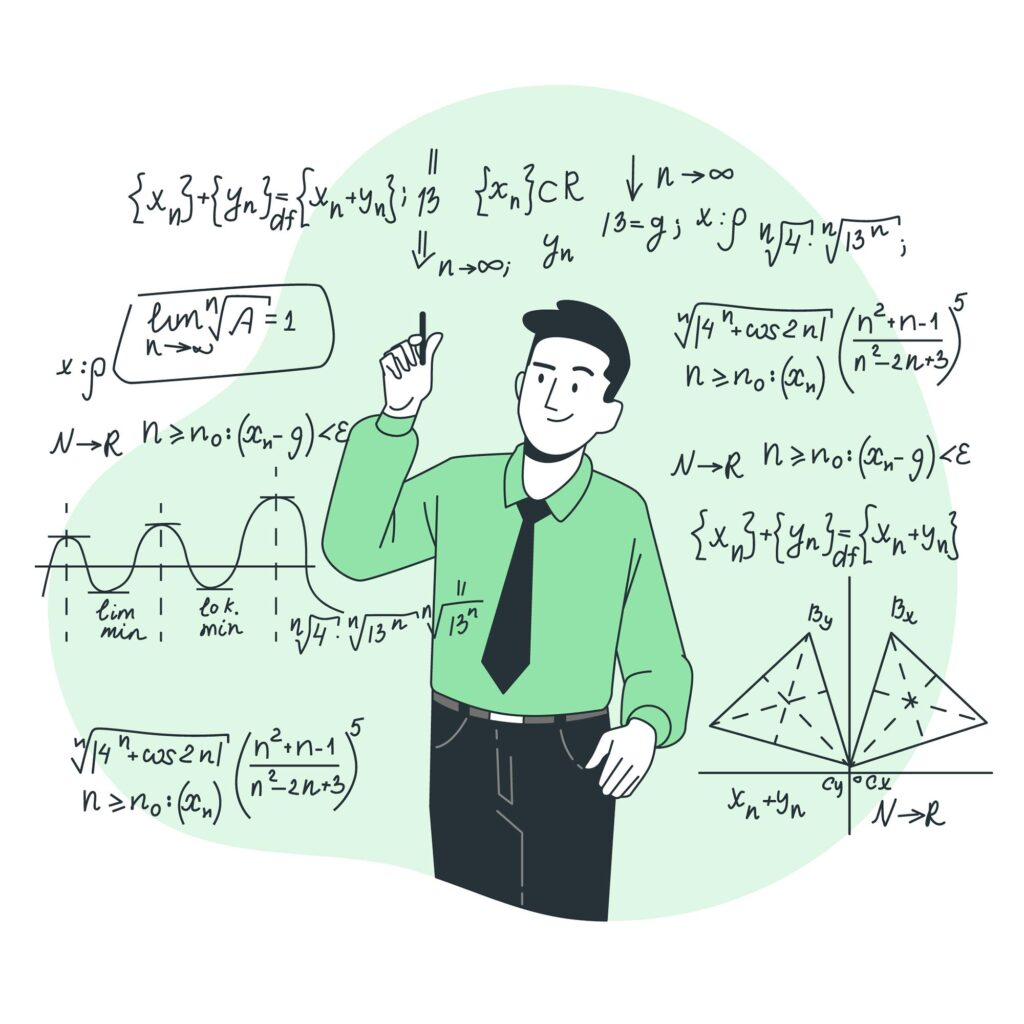## NCERT Maths Class 10th Solution

In this section, we shall study the NCERT Maths Class 10th solution in English. In Maths class 10th, there are 15 chapters. The solution is given chapter-wise in PDF format. In each PDF, every question of each exercise is solved in detail. Chapter 1st Real Numbers NCERT maths class 10th solution of chapter 1st is …